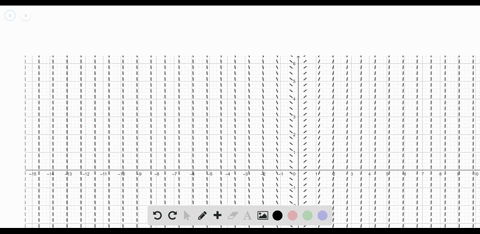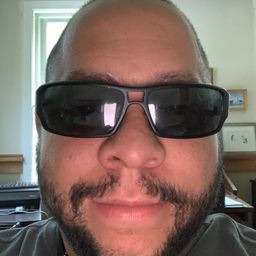Enroll in one of our FREE online STEM summer camps. Space is limited so join now!View Summer Courses### Slope Field In Exercises 47 and $48,$ (a) use a g…

02:43University of Delaware

Need more help? Fill out this quick form to get professional live tutoring.

Get live tutoring
Problem 46

Slope Field In Exercises 45 and $46,$ a differential equation, a point, and a slope field are given. A slope field (or direction field) consists of line segments with slopes given by the differential equation. These line segments give a visual perspective of the slopes of the solutions of the differential equation. (a) Sketch two approximate solutions of the differential equation on the slope approximate of which passes through the indicated point. (To print field, one of which passes through the indicated point. (To print
an enlarged copy of the graph, go to Math Graphs.com.) (b) Use integration and the given point to find the particular solution of the differential equation and use a graphing utility to graph the solution. Compare the result with the sketch in part (a) that passes through the given point.
$\frac{d y}{d x}=-\frac{1}{x^{2}}, x>0,(1,3)$

$$y=\frac{1}{x}+2$$

## Discussion

You must be signed in to discuss.

## Video Transcript

So here we have a slope field frontier. Lex. Thank you. Should be fairly obvious. It's going to go something like, Let's find out why. Yeah. Is it one over X squared? Cheers. Negative x Tonight too, Jax. I can't live my ex too. No, I think they had one divide by that. That's just one X and then wanted to go to the point one cream. This is what that means. Three should be the one three. See, You want to and fly because specs one two. Hopefully, you can see that. That's exactly what's happening here off.